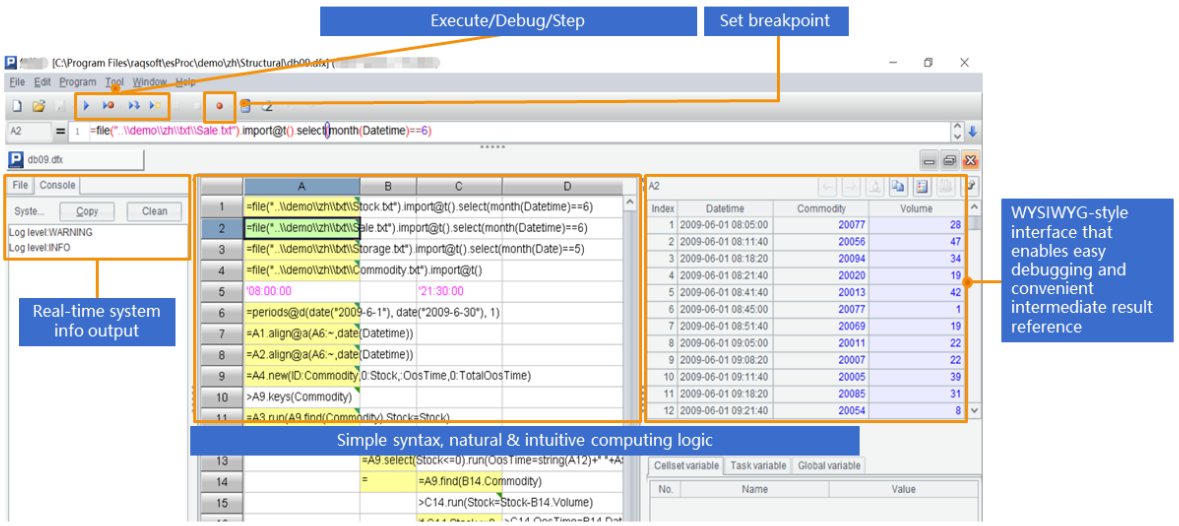# 有没有Java版类似SQLite的开源库？

JAVA调用SPL只需JDBC接口，基本看一遍就会。比如把tab分隔的文本文件Orders.txt按Client列顺序排序，按Amount列逆序排序：

```…
Class.forName("com.esproc.jdbc.InternalDriver");
Connection connection   =DriverManager.getConnection("jdbc:esproc:local://");
Statement statement =   connection.createStatement();

String str="=T(\"D:/Orders.txt\").sort(Client,-   Amount)";
ResultSet result =   statement.executeQuery(str);
…
```

```str="\$select   * from d:/Orders.txt order by Client, Amount desc"
```

```//条件查询
str="=T(\"D:/Orders.txt\").select(Amount>1000   && Amount<=3000 && like(Client,\"*S*\"))";
//分组汇总
str ="=T(\"D:/Orders.txt\").groups(year(OrderDate);sum(Amount))";
//关联
str ="=join(T (\"D:/Orders.txt\"):O,SellerId;   T(\"D:/data/Employees.txt\"):E,EId).new(O.OrderID,O.Client,O.SellerId,O.Amount,O.OrderDate,   E.Name,E.Gender,E.Dept)";```

 A B 1 =[T("d:/orders1.csv"),   T("d:/orders2.csv")].merge@u() /对记录做并集 2 file("d:/fast.btx").export@b(A1) /写入集文件

```str="=T(\"D:/fast.btx\").sort(Client,-   Amount)"

str="\$select   * from d:/fast.btx order by Client, Amount desc"```

btx还可以预先进行有序化处理，从而获得更高计算性能，比如进行二分查找，详情参考官网。

 A B 1 = T("D:/data/sales.csv").sort(amount:-1) 取数并逆序排序 2 =A1.cumulate(amount) 计算累计序列 3 =A2.m(-1)/2 最后的累计值即是总和 4 =A2.pselect(~>=A3) 超过一半的位置 5 =A1(to(A4)) 按位置取值

```        …
Class.forName("com.esproc.jdbc.InternalDriver");
Connection connection   =DriverManager.getConnection("jdbc:esproc:local://");
Statement statement =   connection.createStatement();
ResultSet result =   statement.executeQuery("call bigCustomer ()");
...```

 A B 1 =T("d:/AAPL.xlsx") 读Excel文件，首行为列名 2 =a=0,A1.max(a=if(price>price[-1],a+1,0)) 求最长连续上涨天数`= file("D:/Orders.txt").import@t(;,"||")`

SPL支持多种数据源，除了文本文件，还有Excel、数据库、各类NoSQL、webService、restful等。比如：restful返回多层JSON，用来描述多个员工和员工的多个订单，需要查询出所有价格在500-2000，且客户名包含bro字样的订单。SPL代码如下：

 A 1 =json(httpfile("http://127.0.0.1:6868/api/getEmpOrders").read()) 2 =A1.conj(Orders) 3 =A2.select(Amount>500 &&   Amount<=2000 && like@c(Client,"*bro*"))